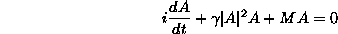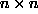The Discrete Self-Trapping Equation

The equationwhereis a complex n-vector, and M is areal symmetric matrix, is known as the Discrete Self-Trapping (DST) Equation. See J. C. Eilbeck, P. S. Lomdahl, and A. C. Scott, Physica D, 16, 318-338, 1985, for details. In the cases where M is tri-diagonal with constant coefficients, this reduces to a discrete form of the Nonlinear Schrödinger (DNLS) equation. A survey concentrating on the quantized version of the DST equation will be found in A. C. Scott, J. C. Eilbeck and H. Gilhoj, Quantum lattice solitons, Physica D 78, 194-213, 1994.

A bibliography file dst.pdf on this equation, is available. Alternatively, download the file dst.tex and the accompanying .bib file dst.bib and process these using LaTeX and BibTeX.

Some old papers

Here you will find some of my older papers on this topic published in obscure conference proceedings which are now difficult to obtain.
Chris Eilbeck / Heriot-Watt University / chris@ma.hw.ac.uk / tel. +44 (0)131 451 3220